TitleCollege Algebra
Tutorial 14: Linear Equations in One VariableLearning Objectives

 After completing this tutorial, you should be able to: Know what a linear equation is. Know if a value is a solution or not. Use the addition, subtraction, multiplication, and division properties of equalities to solve linear equations. Classify an equation as an identity, conditional or inconsistent.Introduction

 This is where we start getting into the heart of what algebra is about - solving equations.  In this tutorial we will be looking specifically at linear equations and their solutions.  We will start off slow and solve equations that use only one property to make sure you have the individual concepts down.  Then we will pick up the pace and mix 'em up where you need to use several properties and steps to get the job done.  Equations can be used to help us solve a variety of problems. In later tutorials, we will put them to use to solve word problems.Tutorial

 Equation Two expressions set equal to each other

 Linear Equation An equation that can be written in the form  ax + b = 0 where a and b are constants

 Note that the exponent (definition found in Tutorial 2: Integer Exponents) on the variable of a linear equation is always 1.

 The following is an example of a linear equation: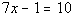Solution A value, such that, when you replace the variable with it, it makes the equation true. (the left side comes out equal to the right side)

 Solution Set Set of all solutions

 Solving a Linear Equation  in General Get the variable you are solving for alone on one side and everything else on the other side using INVERSE operations.

 The following will give us the tools that we need to solve linear equations.

 Addition and Subtraction Properties of Equality If a = b, then a + c = b + c If a = b, then a - c = b - c

 In other words, if two expressions are equal to each other and you add or subtract the exact same thing to both sides, the two sides will remain equal. Note that addition and subtraction are inverse operations of each other.  For example, if you have a number that is being added that you need to move to the other side of the equation, then you would subtract it from both sides of that equation.Example 1:  Solve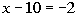for x.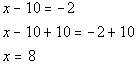*Inverse of sub. 10 is add. 10

 Note that if you put 8 back in for x in the original problem you will see that 8 is the solution to our problem.Example 2: Solve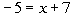for x.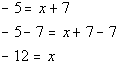*Inverse of add. 7 is sub. 7

 Note that if you put -12 back in for x in the original problem you will see that -12 is the solution we are looking for.

 Multiplication and Division Properties of Equality If a = b, then a(c) = b(c) If a = b, then a/c = b/c where c is not equal to 0.

 In other words, if two expressions are equal to each other and you multiply or divide (except for 0) the exact same constant to both sides, the two sides will remain equal.  Note that multiplication and division are inverse operations of each other.  For example, if you have a number that is being multiplied that you need to move to the other side of the equation, then you would divide it from both sides of that equation.  Note, for multiplication and division, it is not guaranteed that if you multiply by the variable you are solving for that the two sides are going to be equal.  But it is guaranteed that the two sides are going to be equal if you are multiplying or dividing by a constant or another variable that you are not solving for.  We will talk more about this in a later tutorial.  For this tutorial just note you can use this property with constants and variables you are not solving for.Example 3:  Solve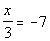for x.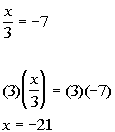*Inverse of div. by 3 is mult. by 3

 If you put -21 back in for x in the original problem, you will see that -21 is the solution we are looking for.Example 4:   Solve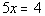for x.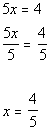*Inverse of mult. by 5 is div. by 5

 If you put 4/5 back in for x in the original problem, you will see that 4/5 is the solution we are looking for.

 The examples above were using only one property at a time to help you understand the different properties that we use to solve equations.  However, most times, we have to use several properties to get the job done.  The following is a strategy that you can use to help you solve linear equations that are a little bit more involved.

 Strategy for Solving a Linear Equation

Note that your teacher or the book you are using may have worded these steps a little differently than I do, but it all boils down to the same concept -   get your variable on one side and everything else on the other using inverse operations.

Step 1: Simplify each side, if needed.

 This would involve things like removing ( ), removing fractions, adding like terms, etc.  To remove ( ):  Just use the distributive property. To remove fractions: Since fractions are another way to write division, and the inverse of divide is to multiply, you remove fractions by multiplying both sides by the LCD of all of your fractions.

Step 2: Use Add./Sub. Properties to move the variable term to one side and all other terms to the other side.

Step 3: Use Mult./Div. Properties to remove any values that are in front of the variable.

I find this is the quickest and easiest way to approach linear equations.Example 5:  Solve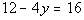for y.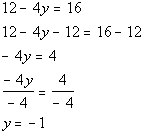*Inverse of add. 12 is sub. 12   *Inverse of mult. by -4 is div. by -4

 Be careful going from line 4 to line 5.  Yes, there is a negative sign. But, the operation between the -4 and x is multiplication not subtraction.  So if you were to add 4 to both sides you would have ended up with -4x + 4 instead of the desired x.  If you put -1 back in for y in the original problem you will see that -1 is the solution we are looking for.Example 6:  Solve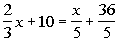for x.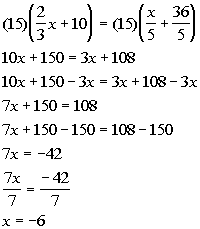*To get rid of those yucky  fractions,  mult. both sides by the LCD of 15   *Get all the x terms on one side   *Inverse of add. 150 is sub. 150   *Inverse of mult. by 7 is div. by 7

 If you put -6 back in for x in the original problem you will see that -6 is the solution we are looking for.Example 7:  Solve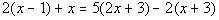for x.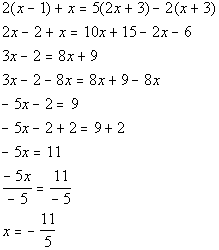*Remove ( ) by using dist. prop.   *Get all x terms on one side *Inverse of sub. 2 is add. 2   *Inverse of mult. by -5 is div. by -5

 If you put -11/5 back in for x in the original problem you will see that -11/5 is the solution we are looking for.

 Identity An equation is classified as an identity when it is true for ALL real numbers for which both sides of the equation are defined.Example 8:   Solve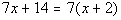for x.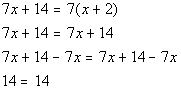*Remove ( ) by using dist. prop. *Get all the x terms on one side

 Where did our variable, x, go???  It disappeared on us.  Also note how we ended up with a TRUE statement, 14 does indeed equal 14.  This does not mean that x = 14.  Whenever your variable drops out AND you end up with a TRUE statement, then the solution is ALL REAL NUMBERS. This means that if you plug in any real number for x in this equation, the left side will equal the right side. Also note that in line 2 above, both sides of the equation have the same expression.  This is another sign that this equation is an identity. So the answer is all real numbers, which means this equation is an identity.

 Conditional Equation A conditional equation is an equation that is not an identity, but has at least one real number solution.Example 9:   Solve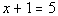for x.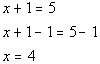*Inverse of add. 1 is sub. 1

 If you put 4 back in for x in the original problem you will see that 4 is the solution we are looking for. This would be an example of a conditional equation, because we came up with one solution.

 Inconsistent Equation An inconsistent equation is an equation with one variable that has no solution.Example 10:   Solve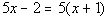for x.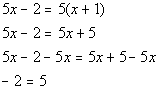*Remove ( ) by using dist. prop. *Get all the x terms on one side

 Where did our variable, x, go???  It disappeared on us.  Also note how we ended up with a FALSE statement, -2 is not equal to 5.  This does not mean that x = -2 or x = 5.  Whenever your variable drops out AND you end up with a FALSE statement, then after all of your hard work, there is NO SOLUTION. So, the answer is no solution which means this is an inconsistent equation.Practice Problems

 These are practice problems to help bring you to the next level.  It will allow you to check and see if you have an understanding of these types of problems. Math works just like anything else, if you want to get good at it, then you need to practice it.  Even the best athletes and musicians had help along the way and lots of practice, practice, practice, to get good at their sport or instrument.  In fact there is no such thing as too much practice. To get the most out of these, you should work the problem out on your own and then check your answer by clicking on the link for the answer/discussion for that  problem.  At the link you will find the answer as well as any steps that went into finding that answer.Practice Problems 1a - 1c: Solve the given equation.

 1a.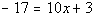(answer/discussion to 1a) 1b.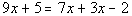(answer/discussion to 1b)

 1c.(answer/discussion to 1c)Practice Problems 2a - 2c: Determine whether the equation is an identity, a conditional equation or an inconsistent equation.

 2a.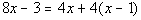(answer/discussion to 2a) 2b.(answer/discussion to 2b)

 2c.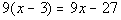(answer/discussion to 2c)Need Extra Help on these Topics?

The following are webpages that can assist you in the topics that were covered on this page:

 http://www.wtamu.edu/academic/anns/mps/math/mathlab/int_algebra/int_alg_tut7_lineq.htm This website helps you to solve linear equations in one variable. http://www.purplemath.com/modules/solvelin.htm This webpage helps you to solve linear equations. http://www.mathpower.com/tut50.htm This webpage gives an example of solving a linear equation. http://www.sosmath.com/algebra/solve/solve0/solve0.html#linear This website helps you to solve linear equations.  Only do the linear equations at this site.  Do not go on to equations containing radicals - that will be covered in a later tutorial.

Go to Get Help Outside the Classroom found in Tutorial 1: How to Succeed in a Math Class for some more suggestions.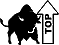Videos at this site were created and produced by Kim Seward and Virginia Williams Trice.
Last revised on Dec. 16, 2009 by Kim Seward.